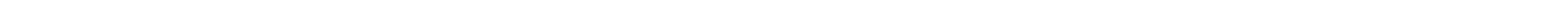3 Acceleration of suprathermal ions at a strong
interplanetary shock

Solar energetic particles events are categorized into two classes, gradual and impulsive events. This classification was introduced by Cane et al. , and further developed by Reames [1990,1995,1999], Kahler [1992,1994], and Gosling . While solar energetic particles in impulsive events originate in solar flares, gradual solar energetic particle events result from particle acceleration at CME-driven interplanetary shocks. Gradual events typically have elemental composition that reflects conditions in the solar corona. The mean charge states of all elements and the Fe/O ratio are similar to those in the solar wind. These events are also called proton-rich because of their large proton-to-electron ratio at high particle energies. The interplanetary particle flux of impulsive events is relatively low, and the heavy ions as well as 3He are enriched relative to their coronal abundances. The mean charge state of Fe is significantly higher, indicating higher temperatures in the source plasma at the Sun. Often events show both signatures. Since many CMEs are accompanied by flares, a combination of the contributions can be expected (e.g. Cohen et al. , Cane et al. ).

The solar energetic particle event associated with the Bastille Day event was one of the strongest in this cycle. The strong interplanetary shock preceeded by a first magnetic cloud was followed by strong magnetic turbulence and a second magnetic cloud. Different processes at and around the shock energize the particles and determine their propagation: (1) stochastic acceleration in the magnetic turbulence, (2) first-order Fermi acceleration at the shock, and (3) spatial diffusion in the field irregularities of the magnetic clouds and in the undisturbed solar wind. In turn, energetic particles also affect the interplanetary plasma, for instance, they amplify waves. Right at the shock the signature of first-order Fermi acceleration, also called diffusive shock acceleration, dominates. Particles are scattered back and forth from the upstream to the downstream plasma and vice versa. During each bounce a particle gains a speed increment of the order of the difference between the upstream and downstream plasma speeds. In its idealized form first-order Fermi acceleration (two homogenous and infinitely extended plasma regions separated by a planar shock) tends to produce energy spectra with a power-law distribution: f(p) = A p, where the spectral index is γ = 3r/(r-1), the shock compression ratio of the densities is r = ndown/nup, and p is the momentum of the particle. The constant A reflects the efficiency of particle injection into the first-order Fermi process.

The HSTOF sensor has a large geometrical factor (0.22 cm2 sr). It has also low background and thus sufficient statistics which results in a good time-resolution. Therefore, the evolution of suprathermal particles can be analyzed with a spatial resolution of approximately ΔL ≈ 0.02 AU. The spectra associated with the Bastille Day event exhibit a complicated evolution as shown in Figure 2. They fit a scheme that orders spectral features by the Q/A (charge-to-mass) ratio of the ion species [Bamert et al., 2003]. Even during the passage of the shock we observe no complete power-laws in particle speed.

The spectra are fitted by a sum of two semi-empirical functions
f = f1 + f2 [Bamert et al., 2003]. The function f1 describes the ions resulting from first-order Fermi acceleration with spectral index γ1 [Forman and Webb, 1985], and an exponential term describing rigidity-dependent escape from the first-order Fermi process [Ellison and Ramaty, 1985]: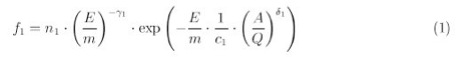The exponential term denotes the propagation of ions in the upstream magnetic cloud. The variables c1 and δ1 depend on the distance to the shock and are related to transport theory of charged particles in solar wind turbulence [Kallenbach et al., 2003].
The function
f2 describes the suprathermal population generated in the strong magnetic turbulence: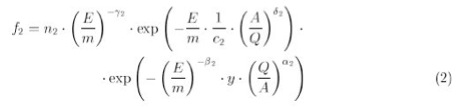The variable parameters c2, δ2, and part of y again describe propagation effects. The constants γ2, β2, and α2 are related to the particular plasma conditions in the downstream turbulence and in the downstream magnetic cloud of the Bastille event, where ions are stochastically accelerated.

The suprathermal particles described by Equation 2 have a maximum in their flux between 60 keV/amu and 1 MeV/amu. This maximum is a function of mass-per-charge and the spatially variable plasma conditions mainly described by the parameter
y (Kallenbach et al., , Eq. 1).
Observations on suprathermal ions associated with the Bastille Day event suggest that the dominant suprathermal particle population is the one trapped in the strong magnetic turbulence between the strong interplanetary shock and the magnetic cloud downstream from the shock.

Detailed quantitative understanding has mainly been achieved for first-order Fermi acceleration. At quasi-parallel shocks, first-order Fermi acceleration requires scattering of the particle in both the upstream and the downstream plasma in order to bounce the particle back and forth across the shock. While the turbulence downstream of interplanetary shocks efficiently scatters ions, the capability of the upstream plasma of scattering ions is less obvious.
We analyzed data within 0.11 AU upstream of the shock [
Bamert et al., 2004a]. We have compared our observations to the predictions of the theory of coupled hydromagnetic wave excitation and ion acceleration at interplanetary traveling shocks by Lee , which is based on quasi-linear theory (QLT).

In the left panels of
Figure 3 proton spectra in the energy range 60--2000 keV derived from HSTOF for four time periods are shown. In the right panels the power spectral densities of magnetic field fluctuations with frequencies between 0.05 mHz and 0.5 Hz for the same time periods are shown. They are calculated from data of the magnetometer MAG onboard ACE. The relative power spectral densityand the omni-directional phase space density of the protons, that amplify the anti-sunward propagating hydromagnetic waves, Fp (k,z) are related by a
growth factor Γ
p (k) k-6 [Lee, 1983]: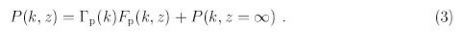where z is the upstream distance to the shock. The phase space density of the protons solves the above equation and the transport equation simultaneously [Lee, 1983]: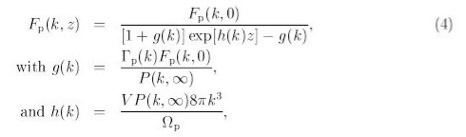where V is the upstream solar wind speed in the shock frame and Ωp the angular gyrofrequency of the protons. Two sets of functions Fp (k,z) and
P(k,z) have been fitted to the data. One applied the theory by Lee  as is (Figure 3, dashed~line). The second set of functions (Figure 3, solid~line) additionally contains a cut-off in γp(k) for k > kc ≈ 3.3 k1MeV, i.e., for Ep > Ec ≈ 90 keV. This cut-off accounts for the enhancement of the observed proton phase-space density for energies below 150 keV (for k < 2.5 k1MeV) above the level predicted by Lee  in the upstream region of the shock. This cut-off factor reflects the fact that the theory of Lee  cannot account for all phenomena of wave-particle interaction in the upstream plasma for protons at energies below 100 keV. Measurements of the full angular distribution of protons in the energy range below 100 keV could improve the understanding of these phenomena.

4 Suprathermal ions of solar and interstellar origin